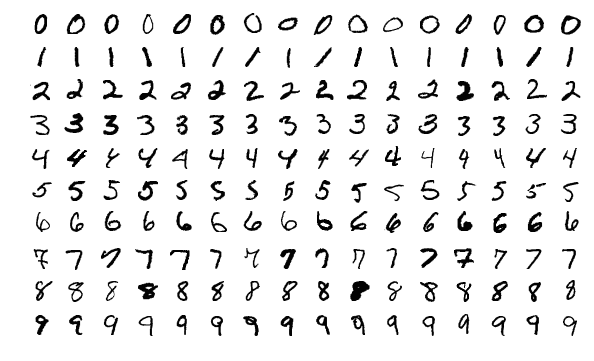## 深度学习：PyTorch 构建神经网络

3984 字 · 160 阅读 · 2023 年 05 月 27 日### 知识点

• PyTorch 构建神经网络
• Sequential 容器结构
• 使用 GPU 加速训练
• 模型保存与推理

PyTorch 搭建神经网络结构的组件在 torch.nn，这些神经网络层大多以类出现，例如全连接层：torch.nn.Linear() ，MSE 损失函数类：torch.nn.MSELoss() 等。

### PyTorch 构建神经网络# 从镜像服务器下载数据集
wget -nc "http://labfile.oss.aliyuncs.com/courses/1081/MNIST.zip"
!unzip -o "MNIST.zip"

import torchvision

# 加载训练数据，参数 train=True，供 60000 条
train = torchvision.datasets.MNIST(
# 加载测试数据，参数 train=False，供 10000 条
test = torchvision.datasets.MNIST(


train.data.shape, train.targets.shape, test.data.shape, test.targets.shape


import torch

# 训练数据打乱，使用 64 小批量
batch_size=64, shuffle=True)
# 测试数据无需打乱，使用 64 小批量
batch_size=64, shuffle=False)


import torch.nn as nn
import torch.nn.functional as F

class Net(nn.Module):
def __init__(self):
super(Net, self).__init__()
self.fc1 = nn.Linear(784, 512)  # 784 是因为训练是我们会把 28*28 展平
self.fc2 = nn.Linear(512, 128)  # 使用 nn 类初始化线性层（全连接层）
self.fc3 = nn.Linear(128, 10)

def forward(self, x):
x = F.relu(self.fc1(x))  # 直接使用 relu 函数，也可以自己初始化一个 nn 下面的 Relu 类使用
x = F.relu(self.fc2(x))
x = self.fc3(x)  # 输出层一般不激活
return x


model = Net()
model


PyTorch 的好处在于无需像 TensorFlow 那样建立会话，所以你可以初始化一个 784 长度的随机值样本传入网络，测试一下输出：

model(torch.randn(1, 784))


loss_fn = nn.CrossEntropyLoss()  # 交叉熵损失函数


def fit(epochs, model, opt):
# 全数据集迭代 epochs 次
for epoch in range(epochs):
# 从数据加载器中读取 Batch 数据开始训练
for i, (images, labels) in enumerate(train_loader):
images = images.reshape(-1, 28*28)  # 对特征数据展平，变成 784
labels = labels  # 真实标签
outputs = model(images)  # 前向传播
loss = loss_fn(outputs, labels)  # 传入模型输出和真实标签
loss.backward()  # 从最后 loss 开始反向传播
opt.step()  # 优化器迭代
# 自定义训练输出样式
if (i+1) % 100 == 0:
print('Epoch [{}/{}], Batch [{}/{}], Train loss: {:.3f}'
# 每个 Epoch 执行一次测试
correct = 0
total = 0
images = images.reshape(-1, 28*28)
labels = labels
outputs = model(images)
# 得到输出最大值 _ 及其索引 predicted
_, predicted = torch.max(outputs.data, 1)
correct += (predicted == labels).sum().item()  # 如果预测结果和真实值相等则计数 +1
total += labels.size(0)  # 总测试样本数据计数
print('============ Test accuracy: {:.3f} ============='.format(
correct / total))

fit(epochs=1, model=model, opt=opt)  # 训练 1 个 Epoch，预计持续 10 分钟


### Sequential 容器结构

model_s = nn.Sequential(
nn.Linear(784, 512),  # 线性类
nn.ReLU(),  # 激活函数类
nn.Linear(512, 128),
nn.ReLU(),
nn.Linear(128, 10),
)

model_s  # 查看网络结构


opt_s = torch.optim.Adam(model_s.parameters(), lr=0.002)  # Adam 优化器
fit(epochs=1, model=model_s, opt=opt_s)  # 训练 1 个 Epoch


### 使用 GPU 加速训练

torch.cuda.is_available()


# 如果 GPU 可用则使用 CUDA 加速，否则使用 CPU 设备计算
dev = torch.device(
"cuda") if torch.cuda.is_available() else torch.device("cpu")
dev


def fit(epochs, model, opt):
for epoch in range(epochs):
for i, (images, labels) in enumerate(train_loader):
images = images.reshape(-1, 28*28).to(dev)  # 添加 .to(dev)
labels = labels.to(dev)  # 添加 .to(dev)
outputs = model(images)
loss = loss_fn(outputs, labels)
loss.backward()
opt.step()
if (i+1) % 100 == 0:
print('Epoch [{}/{}], Batch [{}/{}], Train loss: {:.3f}'
correct = 0
total = 0
images = images.reshape(-1, 28*28).to(dev)  # 添加 .to(dev)
labels = labels.to(dev)  # 添加 .to(dev)
outputs = model(images)
_, predicted = torch.max(outputs.data, 1)
correct += (predicted == labels).sum().item()
total += labels.size(0)
print('============ Test accuracy: {:.3f} ============='.format(
correct / total))


model_s.to(dev)


fit(epochs=1, model=model_s, opt=opt_s)  # 训练 1 个 Epoch


### 模型保存与推理

torch.save(model_s, './model_s.pt')


model_s = torch.load('./model_s.pt')
model_s


# 对测试数据第一个样本进行推理，注意将张量类型转换为 FloatTensor
result = model_s(test.data.reshape(-1, 28*28).type(torch.FloatTensor).to(dev))
torch.argmax(result)  # 找到输出最大值索引即为预测标签


test.targets  # 第一个测试数据真实标签


### 系列文章•